# SSC CGL 2018 Practice Test Papers | Quantitative Aptitude (Day-33)

Dear Aspirants, Here we have given the Important SSC CGL Exam 2018 Practice Test Papers. Candidates those who are preparing for SSC CGL 2018 can practice these questions to get more confidence to Crack SSC CGL 2018 Examination.

[WpProQuiz 3246]

Click “Start Quiz” to attend these Questions and view Explanation

1) The ratio of present age of Arun to that of Bharat is 9 : 10. 8 years ago the ratio of 1/7 of the Arun’s age that time to ¼ of Bharat’s age that time was 1 : 2.

Quantity I: Present age of Arun
Quantity II: The age of Bharat 4 years before

a) Quantity I<Quantity II

b) Quantity I= Quantity II

c) Quantity I> Quantity II

d) Quantity I≤ Quantity II

2) A piece of work is to be completed in 100days, 11 Men are employed to do the work it is found that after 50 days only 1/3 rd work is completed. Now additionally how many more Men are to be employed to work to finish the work in time?

a) 5
b) 6
c) 7
d) 11

3) B and C are equally efficient but the efficiency of A is half of the each B and C. A and B started a work and 3 days later C joined them. If A alone can do the same work in 14 days, then in how many more days the work will be completed?

a) 1
b) 2
c) 3
d) 4.5

4) A Container has 140 liter of mixture of acid and water, in which water is 10%. 30 liter of this mixture was taken out and replaced with “X” liter acid. If the resultant quantity of acid in the mixture was 112 liter, what is the value of X?

a) 11
b) 12
c) 13
d) 14

5) Pooja’s downstream swimming rate is four times of her upstream swimming rate. If she covers 12 km upstream in 2.5 hours, what distance she will cover in 5 hours downstream?

a) 72 km
b) 86 km
c) 96 km
d) 82 km
6) If 1 is added to both the numerator and the denominator of a fraction, it becomes1 /4. If 2 is added to both the numerator and the denominator of that fraction, it becomes 1 /3.The sum of numerator and denominator of the fraction is –

a) 10

b) 8

c) 15

d) 12

7) From a solid cylinder of height 15 cm and radius of the base 8 cm, a cone of same height and same base is removed. The volume of the remaining solid is –

a) 240πcm3

b) 5280 πcm3

c) 640πcm3

d) 360πcm3

8) Simplify: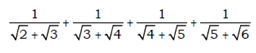a) √3 (√2 -1)

b) √2 (√3 -1)

c) √3−1

d) √2−1

9)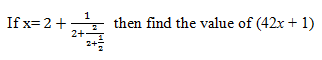a) 45

b) 64

c) 100

d) 80

10) By selling an article at two third of actual selling price, a loss of 20 % is incurred. What is the profit percentage on selling the article at its actual selling price?

a) 20%

b) 30%

c) 25%

d) 40%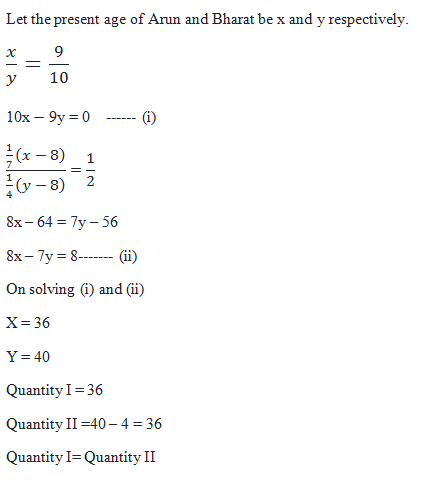11 men complete 1/3 of work in 50 days.

So, 22 men complete 2/3 of work in 50 days

Efficiency of A: B: C = 1:2:2

Let Total work =14

(1+2) × 3 + (1+2+2) x = 14

5X = 14 – 9 = 5

X = 1

Mixture      Acid       Water

140            126           14

After taking 30 liters from the mixture

110             99              11

Replaced with X liter of acid

Quantity of acid after replacement of acid =112

Quantity of X = 112 – 99 = 13

In upstream, Distance= 12 km

Time= 2.5 hours

Upstream Speed= 12/2.5= 4.8 km/hr

Downstream speed= 4(Upstream speed)

Downstream speed= 4(4.8) = 19.2 km/hr

Distance covered in 5 hours downstream= 19.2 x 5 = 96 km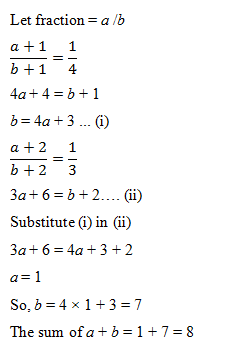The volume of remaining solid = π (8)2 x15 − 1/3xπ (8)2.15

= 640π cm3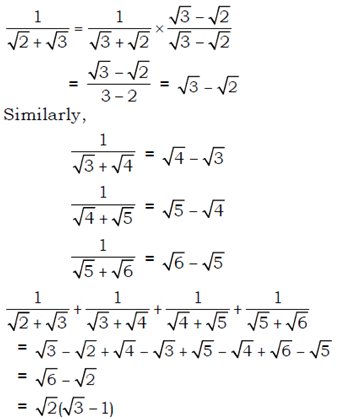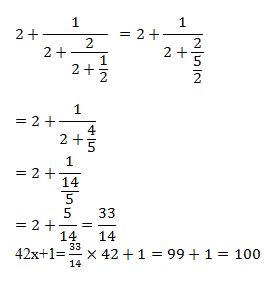Let SP = 300

2/3 of SP = 2/3×300 = 200

A loss of 20% is obtained on SP at 2/3 of SP

CP = 200×100/80 = 250

Profit % on selling at actual selling price= 50/250×100 = 20%